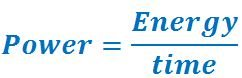# Definition Of Power

## Definition Of Power

"The rate at which the energy is used or produced is called power."
OR The energy used or produced in a second is called power.Power is energy per unit time.
The SI unit of power is Watt.
One watt is one joule per second.
The average power P avg = ∆E/∆t
The unit of power watt is named after the famous English engineer James Watt.
100 watt is called kilowatt and 1000000 (106) watt is called megawatt.

Example 1: A crane lifted a body in three seconds by spending an energy of 1500J. Then the power of crane is 500 watt.
Reason: Power = Energy/time=1500/3=500 watt.0

#### Examples of Power

The power of a generator which generates 1 joule energy in 1 sec is 1 watt.
The energy used by an electric heater of power rating 12 watt is 12 J in a second.

#### Solved Example on Power:

An electric heater generates 12j energy in 2 seconds, and then the power is

E. 1 Watt
F. 3 Watt
G. 6 Watt
H. 12 Watt